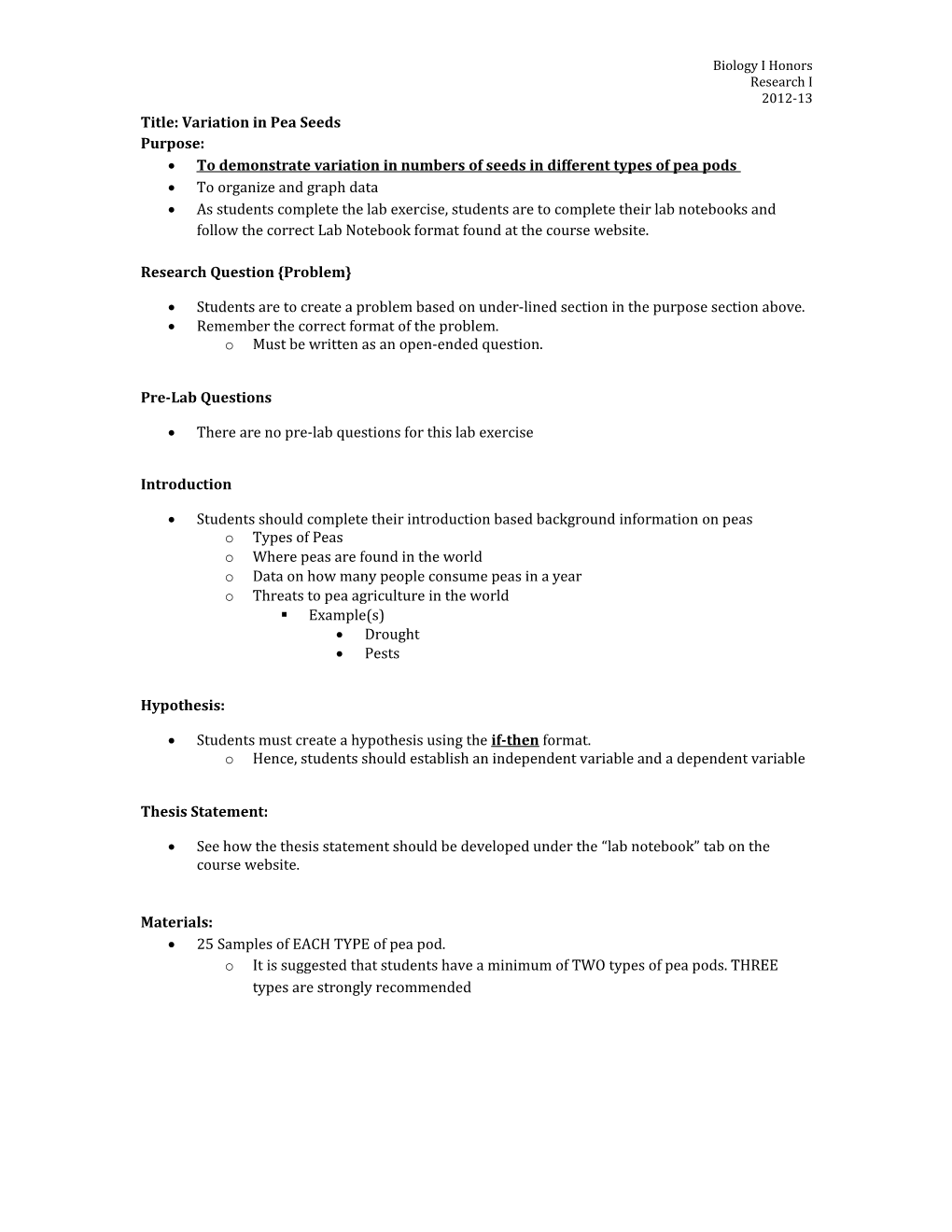# Title: Variation in Pea SeedsBiology I Honors

Research I

2012-13

Title: Variation in Pea Seeds

Purpose:

• To demonstrate variation in numbers of seeds in different types of pea pods
• To organize and graph data
• As students complete the lab exercise, students are to complete their lab notebooks and follow the correct Lab Notebook format found at the course website.

Research Question {Problem}

• Students are to create a problem based on under-lined section in the purpose section above.
• Remember the correct format of the problem.
• Must be written as an open-ended question.

Pre-Lab Questions

• There are no pre-lab questions for this lab exercise

Introduction

• Students should complete their introduction based background information on peas
• Types of Peas
• Where peas are found in the world
• Data on how many people consume peas in a year
• Threats to pea agriculture in the world
• Example(s)
• Drought
• Pests

Hypothesis:

• Students must create a hypothesis using the if-then format.
• Hence, students should establish an independent variable and a dependent variable

Thesis Statement:

• See how the thesis statement should be developed under the “lab notebook” tab on the course website.

Materials:

• 25 Samples of EACH TYPE of pea pod.
• It is suggested that students have a minimum of TWO types of pea pods. THREE types are strongly recommendedString BeansLima BeansProcedure: (Must be written in the correct format in lab notebook…See course website for details)

• Describe the shape, size, texture, color, etc of different types of pea pods
• Open 25 pods of each type of pea
• Count the number of pea seeds in each pod
• Record your data in a data chart
• Determine the following statistics:
• Average number of peas per pod (total number of seeds/total number of pods)
• Range in number of peas per pod
• Median (middle value)
• Mode (most frequent value)
• Obtain data on average number of peas per pod in different types of pea pods from other groups
• Use the averages to construct a bar graph
• Type of seed on horizontal axis
• Average number of seeds on vertical axis

Results:

• Data chart for your pea seed counts
• Data chart for class data
• Graph
• View course website under “Lab Notebook” tab for instructions how to complete results section.

Discussion:

• View course website under “Lab Notebook” tab for instructions how to complete discussion section.

Conclusion:

• View course website under “Lab Notebook” tab for instructions how to complete the conclusion section.
• Include information about which type of pea shows the least variation and which type shows the most variation in number of peas per pod

**STUDENTS ARE TO FOLLOW FORMAT POSTED ONLINE FOR LAB NOTEBOOK.ALL SECTIONS SHOWN ONLINE MUST BE INCLUDED IN LAB NOTEBOOK WRITE-UP. THIS IS A BRIEF CONCISE GUIDE FOR THE LAB EXERCISE.